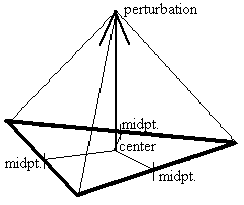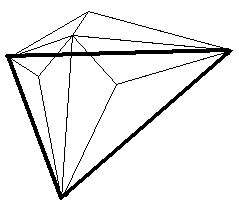# The fractal subdivision algorithm for generating mountains

Markus Altmann

## Overview

The term fractal has been extended beyond Mandelbrot's original definition [FRAC] and include more objects [FOLEY][WATT]. Its precise mathematical definition requires statistical self-similarity at any resolution [FOLEY]. This means parts of fractal objects that look like, but never exactly like parts at different scales [PEIT]. Thus, true fractal objects are only generated by infinite recursive processes. Therefore, those objects generated by a finite number of recursions may exhibit no visible change in detail after some stage, but are adequate approximations of the ideal [FOLEY].

The principle of the subdivision method is to recursively subdivide (split) polygons of a model up to a required level of detail [WATT]. At the same time the parts of the split polygons will be perturbed. The initial shape of the model is retained to an extent, depending on the perturbations. Thus, a central point of the fractal subdivision algorithm is perturbation as a function of the subdivision level [WATT]. Concerning mountains, the higher the level the smaller the perturbation, otherwise the mountains would get higher and higher. In addition there must be a random number generator to obtain irregularities within the shape - and to achieve a kind of statistical similarity:

p_n = P( n ) * RND();

where p_n = perturbation at level n, P( ) = Perturbation function depending on level n, and RND() = random number generator.

Fournier et al. [FOUR] developed a subdivision algorithm for a triangle. Here, the midpoints of each side of the triangle are connected, creating four new subtriangles [WATT] (Fig. 1).Fig. 1: Principle of Fournier subdivision algorithm; perturbation of midpoints of each triangle side (right part) [FOLEY].

For the perturbation of these points, they use the self-similarity and conditional expectation properties of fractional Brownian motion [WATT][PEIT]. From this method, two problems emerge, which are categorized by Fournier et al. as external and internal consistency problems.

Internal consistency requires that the final shape is independent of the orientation of the subdivided triangle. This is satisfied by a Gaussian random number generator dependent on the point's position, generating the same numbers in the same order at a given subdivision level.

The external consistency concerns midpoint displacement at shared edges and their direction of displacement. The first part is already covered by the Gaussian random number generator. The direction problem can be handled by perturbing the midpoint along a normal, which is the average of the polygon normals contributing to the edge. Instead of calculating the average normals at each subdivision, a more practical and cheaper way is to take the normal of the original triangle, as it is done in the following simplified algorithm.

The simplified algorithm also calculated the midpoints, but they are used to get the center of the triangle. Thus, during recursive subdivisions, there is no need to take care of shared edges and points on them, since only this center is displaced (Fig. 2).Figs. 2 (left) and (right): Principle of simplified algorithm. Using midpoints of triangle sides to get center and perturb it (left). Right image shows subtriangles after the second subdivion. Bold lines are sides of original triangle to subdivide.

The degree of perturbation of the center is controlled by an initial displacement, a random number generator of the computer system and a given displacement factor [0 .. 1]. The displacement factor (< 1) is always multiplied with the actual perturbation at each subdivision level, lowering the perturbation at each level. If this factor is equal to 1.0 then only the random number generator takes control over the perturbation due to multiplication with actual perturbation.

A visual defect that is inherent with the above mentioned methods is 'creases' [WATT]. This results from aligned subdivision edges and is noticeable, particularly from a high view point. A practical solution to diminish the creasing problem is to hide the mountain terrain with fog, which also allows one to stop subdivision at a lower level.

## Conclusion

Besides methods of generating mountain terrain out of 3D fractals [3DFRAC], the subdivision algorithm provides a more realistic and flexible way to compute mountain structures. The simplified algorithm might not create mountains as realistic as the algorithm of Fournier et al. since it mainly creates peaks out of a triangle. In addition, because only the center of the triangles are perturbed, one edge of the subtriangles always points to the original vertices of the first subdivision of the original triangle. This results in clear creases - therefore, there should be a lot of fog. However, this algorithm is easy to implement - otherwise I wouldn't call it simplified - and fast, because no special considerations about degree and orientation of displacements at shared edges have to be taken into account.

## References

[FRAC] html page http://www.cis.ohio-state.edu/hypertext/faq/usenet/fractal-faq/faq.html

[3DFRAC] html page http://www.cis.ohio-state.edu/hypertext/faq/usenet/fractal-faq/faq-doc-20.html for 3D fractal images see html pages: http://www.cnam.fr/fractals/mandel3D.html http://www.cnam.fr/fractals/paolo3D.html

[FOLEY] James D. Foley et al., "Introduction to computer graphics", 1994, Addision-Wesley.

[FOUR] A. Fournier, D. Fussell, L. Carpenter, "Computer Rendering of Stochastic Models", June 1982, Comm. ACM, 25(6), pg. 371 - 384.

[PEIT] Heinz-Otto Peitgen, Dietmar Saupe, contributions by M. F. Mandelbrot et al., "The Science of fractal images", 1988, Springer Verlag, New York.

[WATT] Alan Watt, Mark Watt, "Advanced animation and rendering techniques". 1992, AMC press, New York, Addision-Wesley.

matt@owl.WPI.EDU